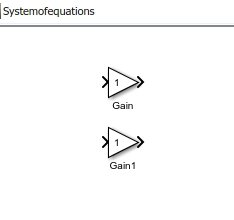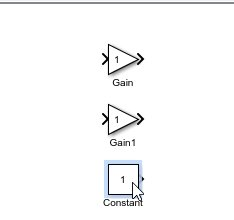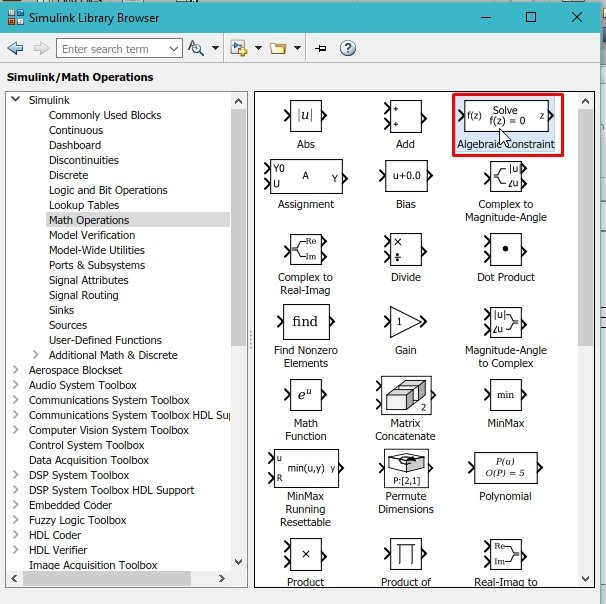# Solving linear equations with Simulink : tutorial 4

In this tutorial, a block diagram is designed which will help us to solve a system of linear equations using MATLABs’ Simulink. At the start a brief introduction to a linear equations and system of linear equations are provided. After that a simple example is performed on MATLAB simulink in which a sample system of linear equations is taken and I solved for the variables involved with the help of simulink. The example taken here is to solve two linear equations for two unknowns we can however extend it for solving larger number of equations simultaneously. At the end a simple exercise is provided regarding the concepts and blocks used in this tutorial.

### Introduction to linear equation and system of linear equations

A linear equation is a mathematical term which is in the form of unknown variables whose power is one and which can be written in the form of point slope equation as given below,

y=mx+c

Where m is the slope of the line and c is the y-intercept. The graph of a linear equation is a straight line. A system of linear equations is, however, a set of linear equations which contains same variable. The number of equations is equal to the number of unknowns present in the equation. For instance, if the system contains 5 linear equations then the number of unknown or variables in all these equations collectively is also 5 with each equation satisfying the definition of linear equations (having the power of each variable equal to 1). In this tutorial we are going to solve a system of linear equations with two equations and two unknowns and the equations are given below,

2x+3y = -4

4x+5y = -5

Lets’ suppose the general system was

a1*x + b1 * y = -k1

a2*x + b2 * y = -k2

This implies that a1 = 2, b1 = 3, k1 = 4, a2 = 4, b2 = 5, k2 = 5

These values will be used in the tutorial later.

### How to solve linear equations with Simulink

Lets’ now move towards a simple example for solving the system of linear equations using Simulink.  In Simulink a block named as Algebraic Constraint will help us do the job for us. However, it is not that simple we also have to apply some logic in order to solve the system of linear equations. Lets’ now begin with the programming part. Open MATLAB and then Simulink as we have done in previous tutorials. After that open the library browser and from the library browser select the commonly used blocks as we have been doing in previous tutorials. From that section select the gain block and drag and drop that gain block on the simulink block diagram section. Place two such gain blocks as the example we have taken here is for two unknown variables, as shown in the figure below,Figure 1: Gain blocks

• These gain blocks are used to enter the value of the co-efficient of each of the variables, hence the number of gain blocks is equal to the number of unknowns in the equation. As we are using a system of equations with two unknowns hence the number of gain blocks is two.
• Next comes the constant part. The constant at the right hand side of the equation can be placed in the block diagram in the form of a constant block. From the commonly used blocks section in the library browser of simulink, select the block of a constant and place it with the two already placed gain blocks as shown in the figure below,Figure 2: Constant block

Figure 3: Math operations

Figure 5: List of signs

• This is the adder of equation 1 so we will name the gain and constant as a1, b1 and k1. The next step here is to place an Algebraic constraint block. This constraint block inputs a function whose input should be equal to zero and estimates the output of the functions value. The output of the block must be provided to the input co-efficient through a feedback loop and with each iteration the output value will be updated until we receive a correct value for that unknown. The initial value provided to the constraint block is zero here. From the math operations section select the Algebraic constraints block as shown in the figure below,Figure 7: Algebraic constraints

Figure 8: Sinks

Figure 9: Display

Figure 10: Block diagram of one equation

Figure 11: Block diagrams for both equations

Figure 12: X value

Figure 13: Y value

Figure 14: Command window

Figure 15: Variable value assignment

Figure 16: Workspace

Figure 17: Output

Exercise:

• Design a block diagram that will be able to solve three linear equations with three unknowns as provided in the equations given below,

3x+4y-2z=9 ;  5x+2y+z=0 ; x-y+5z=11